Section B

# An IoT Data Clustering Algorithm for Efficient and Cost-Effective Human Resource Assessment

Qiong Wu1,*, Lijun Chen1, Ying Shen2
1Office of Human Resource, Changchun University of Technology, Changchun, China, wuqiong@ccut.edu, chenliju@ccut.edu.cn
2School of Economic and Management, Changchun University of Technology, Changchun, China, shenying@ccut.edu.cn
*Corresponding Author: Qiong Wu, +86-043185716147, wuqiong@ccut.edu.cn

© Copyright 2023 Korea Multimedia Society. This is an Open-Access article distributed under the terms of the Creative Commons Attribution Non-Commercial License (http://creativecommons.org/licenses/by-nc/4.0/) which permits unrestricted non-commercial use, distribution, and reproduction in any medium, provided the original work is properly cited.

Received: Apr 12, 2023; Revised: May 06, 2023; Accepted: May 10, 2023

Published Online: Jun 30, 2023

## Abstract

Information and communication technology development has made human resource assessment a top concern for enterprises. However, a key challenge with human resource assessment is adapting to the rapidly changing environment and utilizing various data types for decision-making. Unfortunately, the transmission latency of human resource assessment data in cloud computing platforms can negatively impact user experience. The emergence of mobile edge computing provides a new option for computing-intensive tasks like human resource assessment. A new objective function has been proposed to optimize the task offloading strategy, along with a mathematical model that utilizes the deep recurrent Q-network (DRQN) algorithm to leverage multiple Internet of Things (IoT) nodes. As the amount of human resource assessment data collected by IoT nodes continue to grow, extracting the potential laws and assisting human resources in making informed decisions is increasingly important. A fuzzy weighting clustering algorithm has been proposed to address the difficulty of determining the initial cluster number and center in data clustering based on soft subspace combined with a competitive merging mechanism. Simulation results demonstrate that the DRQN algorithm performs well in terms of energy consumption, cost, and latency, proving that the data collected by IoT nodes can meet the needs of human resource assessment and management after real-time processing. The proposed data clustering algorithm also accurately clusters human resource assessment data, meets high-dimensional data clustering needs, and has significant practical application value.

## I. INTRODUCTION

With the changes in the economic situation, people have entered the era of the knowledge economy. In all development thinking dominated by intelligence, human resources have become the focus of competition for enterprises. Human resource assessment evaluates the skills, abilities, knowledge, and performance of employees or job candidates to determine their suitability for an organization’s job or role. It can involve various methods, such as interviews, skills tests, personality assessments, and performance evaluations. Human resource assessment is an integral part of the recruitment and selection process, as well as ongoing performance management and career development. By assessing the strengths and weaknesses of individual employees, employers can identify areas for improvement, provide targeted training and development opportunities, and make informed decisions about promotions, transfers, and other career advancement opportunities. Effective human resource assessment can help organizations to build a strong and highly skilled workforce, improve employee productivity and retention, and achieve their business goals more effectively. Behind the competition, how to comprehensively manage and assess these human resources is considered the key to improving the efficiency of human resources. Therefore, constructing an artificial intelligence-based human resource assessment is essential in improving the enterprise’s human resource management decision-making, thereby accelerating the optimization of the enterprise’s talent structure [1-2]. Although the assessment includes salary management, training management, personnel management, and other content, to some extent, these contents still need to adapt to the current rapidly changing enterprise environment and competitive environment. Simultaneously, from the functional analysis of the system, many systems still need to be expanded to simple data entry, storage, and query functions, without truly integrating intelligence into the system and using some data mining techniques for employee performance evaluation . In this way, it could be more conducive to the development of employees. As the focus of enterprise development, human resources can be assessed relative to creating a good and fair environment for the majority of enterprise employees and ultimately improving the enthusiasm of employees, which has become the focus and key of current thinking [4-5].

Human resource assessment methods refer to the various techniques and tools used to evaluate employees’ or job candidates’ skills, abilities, knowledge, and performance. Many different methods can be used for human resource assessment, and the choice of method will depend on the specific needs and goals of the organization and the job position being evaluated. Some standard methods used for human resource assessment include interviews, cognitive or aptitude tests, personality assessments, performance evaluations, assessment centers, skills tests, and 360-degree feedback. It is important to note that each of these methods has its strengths and weaknesses, and the choice of method should be based on the specific needs and goals of the organization. Additionally, it is essential to ensure that the assessment methods used are fair, reliable, and valid and comply with relevant legal and ethical standards. Human resource assessment criteria refer to the specific standards or benchmarks used to evaluate employees’ or job candidates’ skills, abilities, knowledge, and performance. The criteria used will depend on the specific needs and goals of the organization and the job position being evaluated. Some common criteria used for human resource assessment include job-related competencies, performance metrics, behavioral indicators, personality traits, educational qualifications, and work experience. Most strikingly, it is important to clearly communicate the assessment criteria to candidates or employees and provide feedback on how they are evaluated throughout the assessment process. By using precise and relevant assessment criteria, organizations can more effectively evaluate the suitability and potential of employees or job candidates for a particular role. Many environmental factors can impact the human resource assessment process, creating problems or challenges. Problems in the human resource assessment process environment include bias and discrimination, inaccurate or incomplete data, lack of standardization, legal and ethical issues, lack of training or expertise, and limited resources. By identifying and addressing these environmental problems, organizations can improve their human resource assessment processes’ accuracy, fairness, and effectiveness and make better-informed decisions about recruitment, development, and performance management.

Internet of Things (IoT) nodes can be quickly controlled, allowing real-time feedback and manipulation of collected data without interference from monitoring . For example, it supports automatic data processing of salary changes caused by salary system reform, salary standard adjustment and grading, promotion, and rank promotion. The system automatically adjusts the salary ratio when employees face job changes such as transfer and promotion. Support the establishment of salary, insurance, and welfare accounting rules in the system and automatically calculate the corresponding results according to these rules. With the rapid development and popularization of smart terminals, and the successful deployment of the fifth-generation mobile communication network (5G), the data amount generated by the network is growing exponentially. Therefore, neither terminal devices nor cloud computing can efficiently solve such problems, and mobile edge computing (MEC) comes into being . Although MEC can offload computing to the edge, the ability of edge servers to process data per unit of time is limited. Therefore, it is necessary to design an efficient offloading scheme. Based on overall consideration of latency, energy consumption, and cost, decide whether the task is to be executed locally or offloaded to an edge server to improve the resource utilization of the MEC system. Additionally, the relevance and usefulness of the data collected from IoT nodes may vary depending on the job position and the assessment goals. That being said, there are certain situations where collecting data from IoT nodes can be helpful in human resource assessment. For example, if an employee’s job involves physical activity or heavy machinery operation, sensors on IoT devices could be used to monitor their movements and identify potential safety hazards. Similarly, if an employee’s job involves customer service or sales, data collected from IoT devices such as chatbots or social media could be used to evaluate their communication skills and customer satisfaction levels. Ultimately, the decision to collect human resource assessment data from IoT nodes should be based on careful consideration of the potential benefits and risks and compliance with relevant privacy and data protection regulations.

It is necessary to process the data collected by the IoT nodes in real-time to meet the needs of human resources assessment management . The data collected for human resource assessment can vary depending on the specific needs and goals of the organization and the job position being evaluated. However, some common data types that may be collected during a human resource assessment include (i) Personal information. This includes basic information such as the employee’s name, contact information, and employment history. (ii) Education and training. This includes information about the employee’s educational qualifications and any training or certifications they have received that are relevant to their job. (iii) Work experience. This includes information about the employee’s previous roles, responsibilities, and accomplishments. (iv) Skills and competencies. This includes information about the employee’s technical and soft skills, as well as any specialized knowledge or expertise they have that is relevant to their job. (v) Performance evaluations. This includes information about the employee’s performance on the job, including feedback from supervisors and colleagues, as well as any metrics or KPIs used to measure job performance. (vi) Personality and behavioral traits. This includes information about the employee’s personality, work style, and behavioral traits that may impact their job performance. (vii) Job-specific data. This includes any additional data relevant to the specific job position being evaluated, such as sales figures for a salesperson or customer satisfaction ratings for a customer service representative. By collecting and analyzing these data types, organizations can better understand their employees’ strengths and weaknesses and make more informed decisions about recruitment, training, and performance management. With the development of information and communications technology, data has grown exponentially. How to use the data generated by the IoT nodes and find the required information in the data to serve human resources assessment has become a current research hotspot. Data mining is an effective means of processing massive data. Data mining analyzes and processes specific types of data, discovers potential laws contained in it, and assists people in making correct decisions . Data mining is the focus of data mining to discover the regular information or potential value contained in it by processing data. Data mining methods include supervised learning, association analysis, cluster analysis, feature selection, and extraction [10-11]. Cluster analysis refers to using specific technical means to discover the inherent law of data, and according to the law of the data is scientifically classified. Cluster analysis is an important method and critical data mining process, directly affecting the final result [12-13]. It is one of the critical research contents in the field of data mining at present.

The main contributions of this study are summarized as follows. First, an IoT-driven edge computing task offloading scheme is proposed. Then, a fuzzy weighting clustering algorithm based on soft subspace combined with a competitive merging mechanism is proposed.

The remainder of this paper is organized as follows. Section II presents related studies on the application of IoT in human resource management, task offloading strategies in MEC, and soft subspace clustering. Section III conducts IoT-driven edge computing task offloading. Section IV proposes feature weighting-based human resource data clustering. Subsequently, Section V presents the simulation results and analyses. Finally, Section VI concludes the paper.

## II. RELATED WORK

2.1. Application of IoT in Human Resource Management

Literally, the focus of human resources assessment is on the assessment. However, human resource teams across multiple industries are leveraging the IoT to improve employee health, safety, productivity, and comfort. In human resource management, the human resource manager supervises and enhances the relationship between employees, from hiring to firing. They must hire the right person for their enterprises to close the skills gap between employees, ensure the safety and comfort of their employees, and provide them with the necessary services and benefits [14-16]. Human resource managers must constantly monitor and track employee work, behavior, activities, and comfort. Traditionally, human resource managers monitor employees by observing them, using feedback forms, or integrating monitoring software into their computers. However, this monitoring method needs to provide accurate and objective assessments, which can lead to better communication and decreased employee engagement . Therefore, such consequences can be avoided by using the IoT in human resource management.

IoT in human resource assessment can help human resource managers perform almost all recruiting, monitoring, or paying duties [18-20]. Only in a few cases does the human resource manager get honest feedback. Even so, it was achieved through much hard work. Getting honest feedback is vital for human resource leaders to take steps to improve the overall workplace and workforce. IoT devices can reduce the burden on human resource specialists while collecting feedback.

2.3. Soft Subspace Clustering

The soft subspace clustering algorithm obtains the importance of each data feature by weighting the individual features in each data cluster to find the subspace where the features with large weights are located . Compared with the hard subspace clustering algorithm, people pay more and more attention to the soft subspace clustering algorithm because of its better flexibility and adaptability to data processing. The soft subspace clustering algorithm is integral to the cluster analysis algorithm. It distinguishes the importance of features by assigning weighting coefficients to different data features and realizes flexible control of the clustering results. The key factors that affect the performance of the soft subspace clustering algorithm include the number of data clusters and the initial clustering center . An excellent clustering algorithm should be able to converge to a reasonable number of clusters, and the initial selection of clustering centers has little influence on the clustering results. Currently, popular clustering methods include data cluster evaluation criteria, cluster visualization, and fuzzy clustering methods. Although these methods can obtain a reasonable number of clusters, considering various data features must be more comprehensive.

The model built in this study is the multi-edge server model of the IoT nodes, which is the task offloading scenario of edge computing. As shown in Fig. 1, the model includes N edge servers (ES) and M smart devices (SD), where ES is deployed on the base station, and SD transmits data through a wireless link. Tasks on SD can be executed locally or on ES based on the latency and power consumption requirements.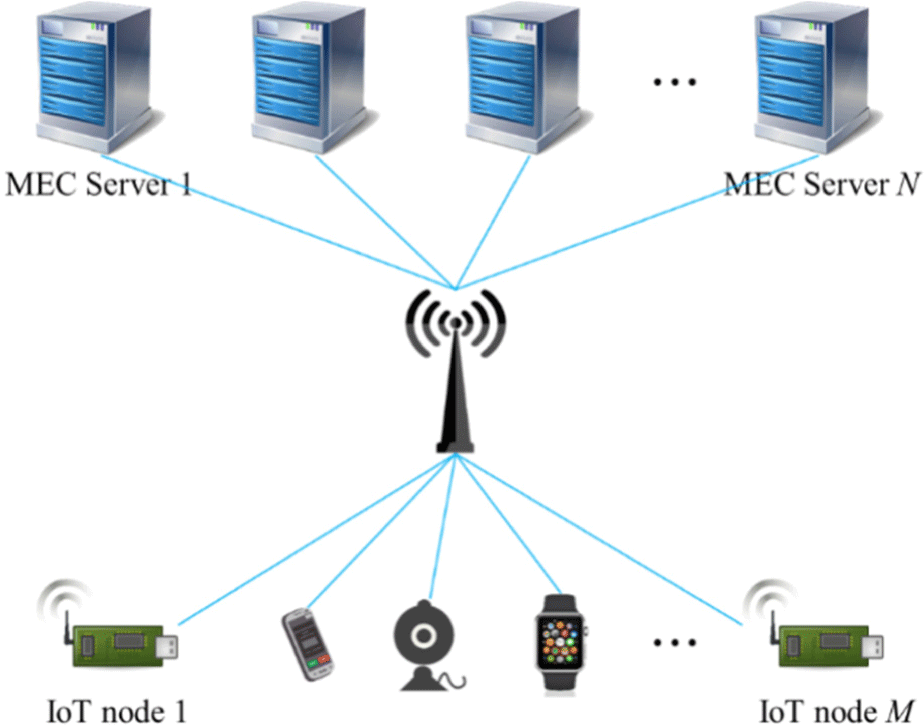Fig. 1. Network model diagram.

Given task granularity , this study adopts fine-grained task partitioning regarding resource utilization and computational complexity. Each task on the IoT nodes can be divided into k subtasks, and different subtasks can be executed on different ES or locally, thus reducing the computing latency. The task set is defined as $R,{R}_{ij}\triangleq \left({D}_{ij}^{in},{C}_{ij},{D}_{ij}^{out},{\omega }_{ij}^{max}\right)$, where i ≤ M, j ≤ k. ${D}_{ij}^{in}$ represents the data amount entered by the jth subtask on the ith IoT node, including codes and input parameters. Cij represents the computing resources required to execute the ijth subtask. Here, the computing resource refers to the CPU. It is assumed that the computing resource required to execute the task on the CPU of the local IoT node is the same as that required to execute the task on the CPU of the ES. ${D}_{ij}^{\text{out}}$ represents the result data obtained after completing the ijth subtask computing, and ${\omega }_{ij}^{\text{max}}$ represents the maximum latency constraint for executing the ijth subtask.

$X=\left(\begin{array}{ccc}{x}_{11}& \cdots & {x}_{1k}\\ ⋮& \ddots & ⋮\\ {x}_{M1}& \cdots & {x}_{Mk}\end{array}\right).$
(1)

If x ∈ {0,1,2,⋯,p,⋯,N}, x = 0 indicates that the subtask is executed locally. If x = 1, the subtask will be offloaded to ES whose number is 1. Similarly, x = p indicates that the subtask will be offloaded to ES whose number is p.

Assuming that the latency of the local processing task is generated only by the CPU, which is defined as follows.

${T}_{ij}^{SD}=\frac{{C}_{ij}}{{f}_{i}^{SD}},$
(2)

where Cij is the computing resource required by Rij. Specifically, it is the number of CPU clock cycles required to execute Rij, and ${f}_{i}^{SD}$ represents the CPU clock speed of IoT node i.

Suppose Rij needs to be offloaded to the ES for execution. In that case, it is necessary to analyze the latency on the ES from three aspects: sending latency, transmission latency, and processing latency, defined as follows.

${T}_{ij}^{ES}=\frac{{D}_{ij}^{in}}{{v}_{i}^{SD-up}}+2\frac{{l}^{SD-ES}}{{v}^{trans}}+\frac{{C}_{ij}}{{f}_{p}^{ES}}+\frac{{D}_{ij}^{out}}{{v}_{p}^{ES-up}},$
(3)

where ${v}_{i}^{\text{SD}-\text{up}}$ is the upload speed of IoT node i, ${v}_{p}^{\text{ES}-\text{up}}$ is the upload speed of edge server p, vtra is the transmission speed on the channel, lSD−ES is the length of the channel between the IoT node and the edge server, ${f}_{p}^{\text{ES}}$ is the CPU frequency of the edge server p, and (2lSD−ES/vtra is the round-trip transmission latency.

When there is the dependency between subtasks, the total latency is the sum of the latency of subtasks executed locally plus the sum of subtasks executed on the edge server.

${T}_{i}={\sum }^{\text{​}}{T}_{ij}^{SD}+{\sum }^{\text{​}}{T}_{ij}^{ES}.$
(4)

When subtasks are independent, the total latency is the maximum latency of all subtasks on the ith IoT node.

${T}_{i}=max\left({T}_{ij}^{SD},{T}_{ij}^{ES}\right).$
(5)

Assuming that the energy consumption generated by tasks executed locally is only related to the CPU, the energy consumption model is defined as follows.

${E}_{ij}^{ES}={p}_{i}^{SD}+{T}_{ij}^{SD},$
(6)

where ${p}_{i}^{SD}$ is the CPU power of IoT node i.

When offloading, the energy consumed by the jth subtask on SDi at the IoT node side is defined as follows.

${E}_{ij}^{ES}={p}_{i}^{SD-up}\frac{{D}_{ij}^{in}}{{v}_{i}^{SD-up}}+{p}_{i}^{SD-free}\left(2\frac{{l}^{SD-ES}}{{v}^{tra}}+\frac{{C}_{ij}}{{f}_{p}^{ES}}+\frac{{D}_{ij}^{out}}{{v}_{p}^{ES-up}}\right),$
(7)

where ${p}_{i}^{\text{SD}-\text{up}}$ is the upload power of the CPU of IoT node i, and ${p}_{i}^{\text{SD}-\text{free}}$ is the discharge power of the CPU of IoT node i when it is idle.

The cost refers to the cost paid to the operator by the user for using the computing resources provided by the operator. Therefore, the cost is only incurred after offloading and does not involve the local execution part. In this study, the cost model based on the remaining computing resources is used; that is, the more computing resources are left, the lower the cost paid by the user, which is defined as follows.

${F}_{ij}={T}_{ij}^{ES}×{u}^{ES}×{R}_{p}^{ES}×To{t}_{p}^{ES},$
(8)

where uES represents the price per unit time of the edge server, ${R}_{p}^{ES}$ represents the utilization rate of computing resources of server p, and $To{t}_{p}^{ES}$ represents the total computing resources of server p.

IoT nodes’ edge computing task offloading problem is an optimization problem. The goal of this study is to minimize energy consumption and cost under the premise of guaranteeing latency and improving the resource utilization rate of the MEC system while extending the battery life of IoT nodes. The objective function is defined as follows.

$\left\{\begin{array}{c}ℒ=\beta \sum _{i=1}^{M}\sum _{j=1}^{k}{E}_{ij}+\epsilon \sum _{i=1}^{M}\sum _{j=1}^{k}{F}_{ij}\\ \underset{X}{\mathrm{min}}\left(ℒ\right)\\ {T}_{ij}\le {\omega }_{ij}^{max}\end{array},$
(9)

where β and ε are the weights of energy consumption and cost, respectively.

3.2. Model Solving

This study proposes a partially observable and fully cooperative edge computing task offloading algorithm based on deep reinforcement learning to solve the above model. In fact, due to the instability of the underlying physical link, the state of the edge server cannot be observed entirely, which is called partially observable . In the reinforcement learning of IoT nodes, each IoT node is autonomous and interactive, which independently receives the status information of the edge server and decides the current offloading scheme according to its policy function. At the same time, IoT nodes communicate with each other to jointly optimize the target value, and a comprehensive standard judges the overall offloading effect.

Markov decision process (MDP) generally contains state space (S), action space (A), and reward function (R) . The state space S is defined as follows: resource surplus vector U, decision matrix X, and objective function .

$S=\left[U,X,G\right],$
(10)

where U = [u1, u2,⋯up,⋯,uN], and up represents the computing resources available to the ES with number p.

The action space A represents all actions that can be taken in each state, which is defined as follows.

$A=\left[i,j,\gamma \right],$
(11)

where λ is the offloading scheme of the jth subtask on the IoT node i, and λ ∈ {0,1,2,⋯,p,⋯,N}.

The reward function R represents the reward obtained by the IoT nodes according to the environmental feedback after performing actions and state transitions, which is defined as follows.

$R=\frac{{ℒ}_{t}-{ℒ}_{t+1}}{{ℒ}^{SD}},$
(12)

where t is the objective function value in period t, t+1 is the objective function value in period t+1 after the IoT node makes the response action At in state St, and SD is the objective function value when the task is executed locally. The above three objective function values are calculated using equation (9). It should be noted that when executed locally, the objective function does not have a cost part (equal to zero), only an energy consumption part and latency constraint. When t+1 > t, that is, after the action At is executed, becomes large; that is, the energy consumption and cost increase, which develops in the undesired direction and gives a negative reward. Conversely, a positive reward is given.

Deep recurrent Q-network (DRQN) is an improvement to deep Q-network (DQN), which uses a recurrent neural network (RNN) to solve partially observable MDP (POMDP) to improve DQN performance. In the MEC application scenario, due to the instability of the underlying physical link and the limitations of the IoT node’s perception, it is difficult for the IoT node to receive accurate state information of the environment in the current period, leading to the POMDP problem . DRQN retains the previous state information, supplements the current state information, and solves the problem of missing values. The specific implementation process is to replace the first fully connected layer after the convolutional layer in DQN with a long short-term memory network and RNN. The DRQN architecture is shown in Fig. 2.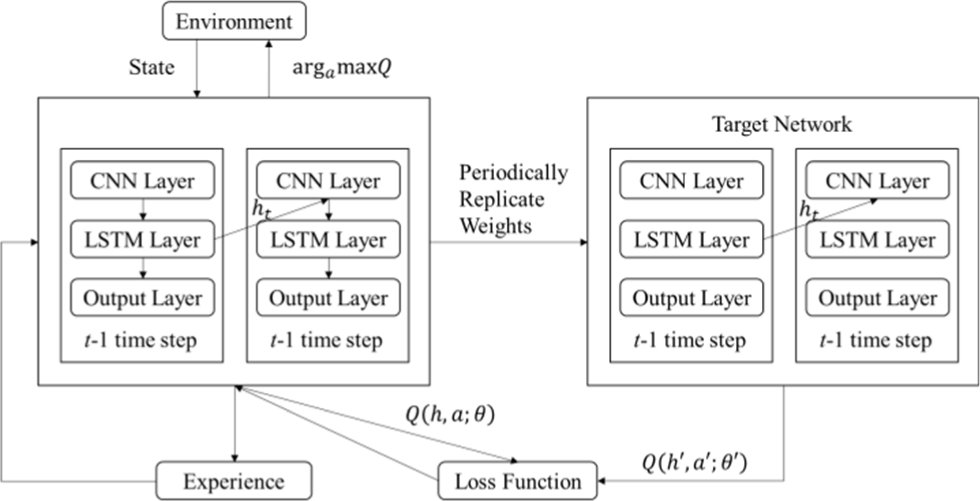Fig. 2. DRQN architecture diagram.

The state information returned by the edge server ES is used as input. The convolutional layer performs convolution operations on the state information to obtain a feature map, which is transferred to the LSTM layer. LSTM supplements the state information according to the previous memory, updates the memory, and finally outputs the Q value through a fully connected layer. Here, ht is the return value of the network at the previous time step, that is, ht = LSTM (ht™1, at), which is used as the input for the next time step.

DRQN uses a neural network with a weight of θ to approximate the Q function, that is, Q(ht,at;θ)≈Q*(st,at). The loss function is the mean square error between the target value and the predicted value, which is defined as follows.

$ℒ={\left(r+\gamma ma{x}_{{a}^{\prime }}Q\left(h\text{'},a\text{'};\theta \text{'}\right)-Q\left(h,a;\theta \right)\right)}^{2},$
(13)

where r is the reward, γ is the discount factor, between 0 and 1, determining the importance of immediate and future rewards. When γ close to 0, immediate rewards are more important. When γ close to 1, future rewards are more important. The neural network updates θ by gradient descent to minimize the loss. It should be noted that the parameter of the target Q is θ′, which is differs the parameter θ of the prediction Q. DRQN uses an independent network called the target network to calculate the target value.

The IoT node executes an action a from the state s to the state s′, and gets a reward r. < s, a, s′, r > is an experience of the IoT node, which is stored in the experience cache. DRQN randomly selects a batch of training samples from the experience cache to reduce the correlation between experiences and improve the algorithm’s generalization.

## IV. FEATURE WEIGHTING-BASED HUMAN RESOURCE DATA CLUSTERING

Human resource assessment data can come in various formats and have different characteristics depending on the specific assessment method, mainly including (i) Quantitative data. It can be expressed numerically, such as test scores, ratings on a scale, or performance metrics. It also can be analyzed using statistical methods to identify patterns or trends. (ii) Qualitative data. It is based on subjective observations or descriptions, such as colleague feedback or open-ended interview responses. Qualitative data can provide valuable insights into employee attitudes and perceptions. (iii) Objective data. It is based on observable, measurable facts, such as attendance records or sales figures. Objective data is often used to evaluate job performance. (iv) Subjective data. It is based on personal opinions or judgments, such as performance ratings or personality assessments. Subjective data can be more difficult to analyze objectively but can provide valuable information about employee potential. (v) Structured data. It is collected using standardized methods like pre-designed assessment tools or surveys. Structured data can be easier to analyze and compare across employees or job positions. (vi) Unstructured data. It is collected through informal or non-standardized methods, such as informal feedback or observations. Unstructured data can provide a more nuanced understanding of employee behavior and performance but can be more challenging to organize and analyze. By understanding the different formats and characteristics of human resource assessment data, organizations can collect, analyze, and use this data more effectively to make informed decisions about employee recruitment, development, and performance management.

Soft subspace clustering, also called feature weighting clustering, refers to assigning weighting coefficients to the features of each data cluster to mark the importance of features in human resource assessment data processing. The competition and mergence mechanism-based fuzzy clustering algorithm uses regularization term to make each clustering center compete and merge. Finally, the number of clusters that meets the requirements is obtained. Merging the soft subspace clustering with the fuzzy clustering of competition and mergence, the fuzzy weighting clustering of soft subspace merged with the competition and mergence mechanism can be obtained. The objective function is defined as follows.

$J=\sum _{i=1}^{c}\sum _{j=1}^{n}{u}_{ij}^{2}\sum _{k=1}^{d}{w}_{ik}^{m}{\left({x}_{jk}-{v}_{ik}\right)}^{2}-rt\sum _{i=1}^{c}{\left(\sum _{j=1}^{n}{u}_{ij}\right)}^{2},$
(14)

where xjk is the human resource assessment dataset, vik is the cluster center, uij is the fuzzy membership degree, wik is the feature weighting coefficient, m is the fuzzy weighting index, rt is the regular term coefficient, c is the number of cluster centers, n is the number of data samples, d is the number of features. The above parameters meet uij ≤ 1, $\sum _{i=1}^{c}{u}_{ij}=1$, and 0 ≤ wik ≤ 1.

Selecting rt reasonably and minimizing J can obtain the number of data clusters and the location of cluster centers that meet the conditions. It uses the Lagrange multiplier method and uses the iterative method to find the vik when J is minimum. The initial number of cluster centers c, cluster centers vik, fuzzy weighting index m and fuzzy membership uij are set according to the requirements, and the iterative process is as follows.

(1) Compute the cluster center vik, the feature weighting coefficient wik, and the potential Pi of the ith data cluster.

${v}_{ik}=\frac{{\sum }_{j=1}^{n}{u}_{ij}^{2}{x}_{jk}}{{\sum }_{j=1}^{n}{u}_{ij}^{2}}.$
(15)
${w}_{ik}=\frac{1}{{\sum }_{l=1}^{d}{\left[\frac{{\sum }_{j=1}^{n}{u}_{ij}^{2}{\left({x}_{jk}-{v}_{ik}\right)}^{2}}{{\sum }_{j=1}^{n}{u}_{ij}^{2}{\left({x}_{jl}-{v}_{il}\right)}^{2}}\right]}^{1}{\left(m-1\right)}}}.$
(16)
${P}_{i}=\sum _{j=1}^{n}{u}_{ij}.$
(17)

(2) Compute the regularization coefficient rt. The regularization coefficient is used to adjust the proportion of the front and back parts of the objective function. It needs to be dynamically computed according to parameters such as the cluster center and weighting coefficient, defined as follows.

$rt\left(t\right)=\frac{\tau \left(t\right){\sum }_{i=1}^{c}{\sum }_{j=1}^{n}{u}_{ij}^{2}{\sum }_{k=1}^{d}{w}_{ik}^{m}{\left({x}_{jk}-{v}_{ik}\right)}^{2}}{{\sum }_{i=1}^{c}{\left({\sum }_{j=1}^{n}{u}_{ij}\right)}^{2}},$
(18)

where τ is the iteration times, τ(t) is the learning factor in each iteration, which is defined as follows.

$\tau \left(t\right)={\tau }_{0}exp\left(-\frac{|t-{t}_{0}|}{\xi }\right),$
(19)

where τ0 is the initial value of learning factor, t0 and ξ are constants selected according to the actual situation.

(3) Compute the fuzzy membership degree uij.

${u}_{ij}={u}_{ij}^{w}+{u}_{ij}^{f}.$
(20)
${u}_{ij}^{w}=\frac{1}{{\sum }_{s=1}^{c}\left[\frac{{\sum }_{k=1}^{d}{w}_{ik}^{m}{\left({x}_{jk}-{v}_{ik}\right)}^{2}}{{\sum }_{k=1}^{d}{w}_{sk}^{m}{\left({x}_{jk}-{v}_{sk}\right)}^{2}}\right]}.$
(21)
${u}_{ij}^{f}=\frac{rt\left(t\right)\left({P}_{i}-\overline{{P}_{j}}\right)}{{\sum }_{k=1}^{d}{w}_{ik}^{m}{\left({x}_{jk}-{v}_{ik}\right)}^{2}},$
(22)

where ${u}_{ij}^{w}$ is the feature weighting distance from the sample to the cluster center. ${u}_{ij}^{f}$ is used to reduce the potential of fake cluster centers in the calculation process. j is the average weighting of the potential of a single sample to the data cluster.

(4) Compute the threshold MT of the data cluster potential and the distance D between each cluster center, and find the minimum value Dmin of the distance D. When the potential Pi of a certain data cluster is less than the threshold MT, the data cluster will be eliminated. When Dmin satisfies equation (24), two data clusters whose distance is Dminare merged. The MT computing and discriminant conditions are defined as follows, respectively.

$MT=\frac{\eta n}{c\left(t\right)}.$
(23)
${D}_{min}<\frac{0.2{\sum }_{r=1}^{R}d\left(r\right)}{Q},$
(24)

where η is the merging threshold parameter, c(t) is the number of cluster centers in tth iteration, d(r) is the distance value between two cluster centers, Q is the total number of distance data between each cluster center.

$Q=\frac{c\left(t\right)\left(c\left(t\right)-1\right)}{2}.$
(25)

Adjust the number of cluster centers c according to the cutting and merging results, and stop the calculation when the number of cluster centers remains stable or meets the iteration end condition, and the obtained vik is the required cluster center result; otherwise return to step (1) and continue.

## V. SIMULATION AND RESULTS ANALYSIS

This study compares DRQN with DQN and random offloading in large-scale heterogeneous clusters in terms of energy consumption, cost, and latency to evaluate the advantages and disadvantages of DRQN in offloading IoT nodes’ human resources assessment tasks . The cluster simulated by the simulation includes 50 edge servers and different numbers of IoT nodes. Among them, the edge servers are evenly distributed in nine areas with the same area, and the IoT nodes are randomly distributed within 150 m from the base station. Assuming that the bandwidth is 20 MHz, the upload speed of the IoT node is 5.3 Mb/s, the upload power is 1,500 mW, the idle discharge power is 200 mW, the limit CPU clock speed ${f}_{\text{max}}^{SD}$ is 2 GHz/s, and the limit CPU frequency ${f}_{\text{max}}^{ES}$ of the edge server is 50 GHz/s. Moreover, the data amount (Kbit) of R follows the uniform distribution of (200, 2000), and the required computing resources follow the uniform distribution of (1200, 3000). When γ is set to 0.7 and 0.9, the energy consumption and cost under different tasks are compared. As shown in Fig. 3, the performance of energy consumption and cost when γ = 0.9 is better than that of 0.7, which means that future reward plays an essential role in edge computing, so γ is set to 0.9 in this study.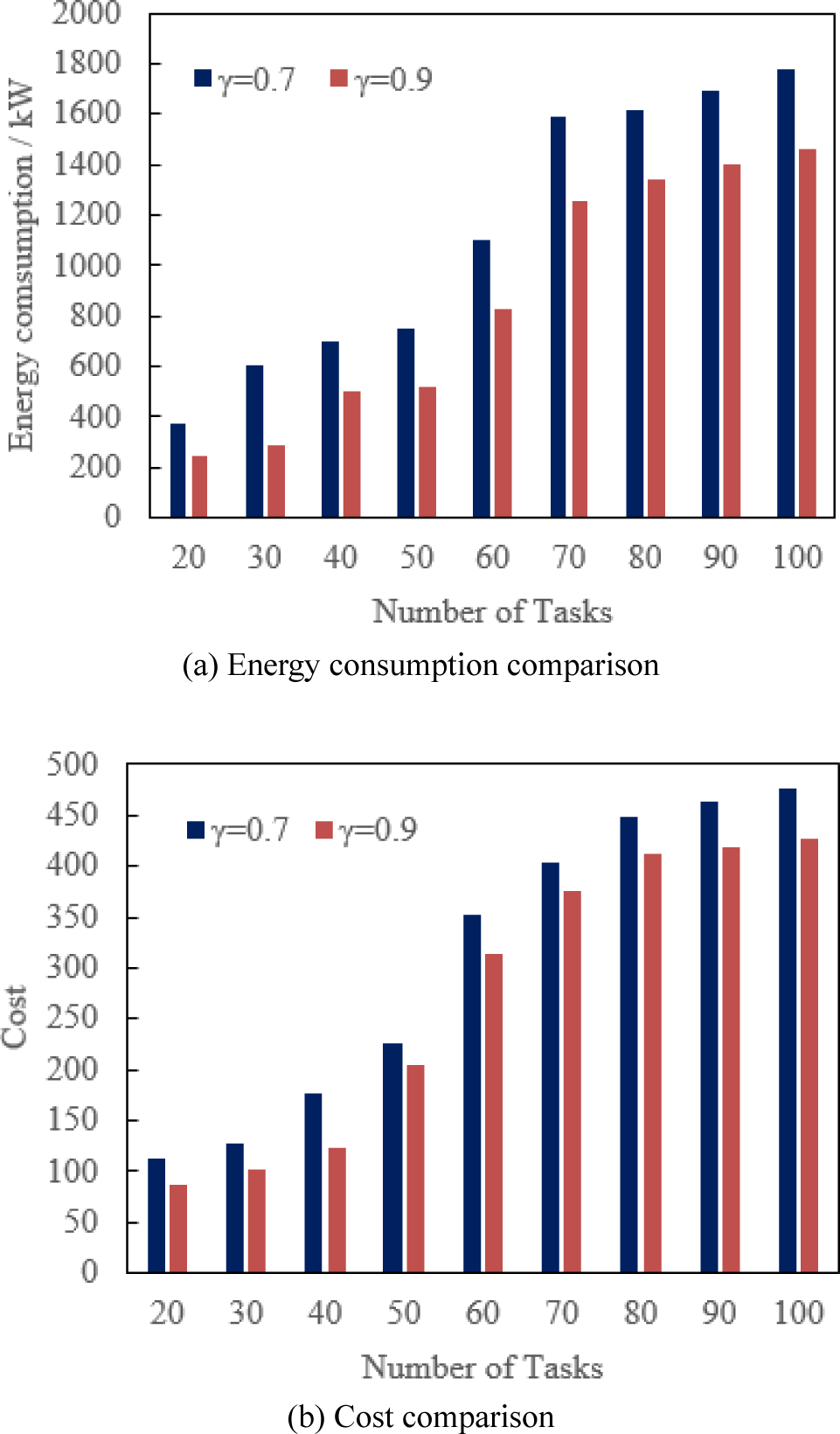Fig. 3. Comparison of energy consumption and cost of DRQN with different discount factors.

To meet the maximum latency, this study takes the energy and cost reduction ratio (ECRR) of the difference between DRQN, DQN, random offloading, and local execution as one of the evaluation metrics of the algorithm. As shown in Table 1, the ECRR of DQN and DRQN reaches more than 50%, and DRQN is nearly two percentage points higher than DQN.

Table 1. Translation performance comparison with other models.
Algorithm ECRR (%)
DQN 52.38
DRQN 54.01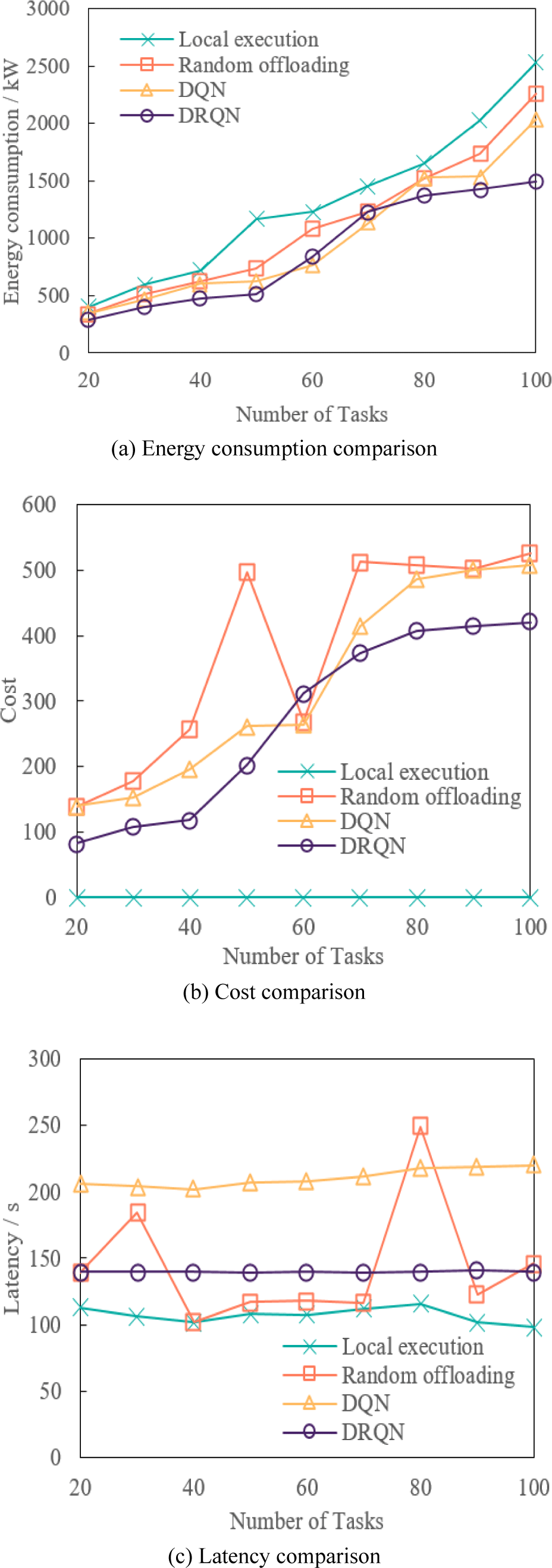Fig. 4. Comparison of simulation results.
5.2. Human Resource Assessment Data Clustering

To intuitively observe the clustering effect of human resources assessment data, three types of Gaussian distribution human resources assessment data are generated in two-dimensional space as the algorithm processes the dataset to verify whether the algorithm can achieve reliable clustering performance. The clustering centers of the three types of human resources assessment data are (20, 60), (40, 40), and (60, 20), respectively. Each class includes 100 data points, and the covariance matrices of the three types are $\left[\begin{array}{cc}5& 0\\ 0& 15\end{array}\right]$, $\left[\begin{array}{cc}8& 0\\ 0& 8\end{array}\right]$, and $\left[\begin{array}{cc}15& 0\\ 0& 5\end{array}\right]$, respectively.

Suppose the initial number of clustering centers is 15, the initial value of learning factor τ0 is 0.7, the time constants t0 and ξ are 10 and 15, the merging threshold parameter η is 0.75, and the fuzzy weighting exponent m is 3. The data points, the true cluster centers and the initial cluster centers are shown in Fig. 5.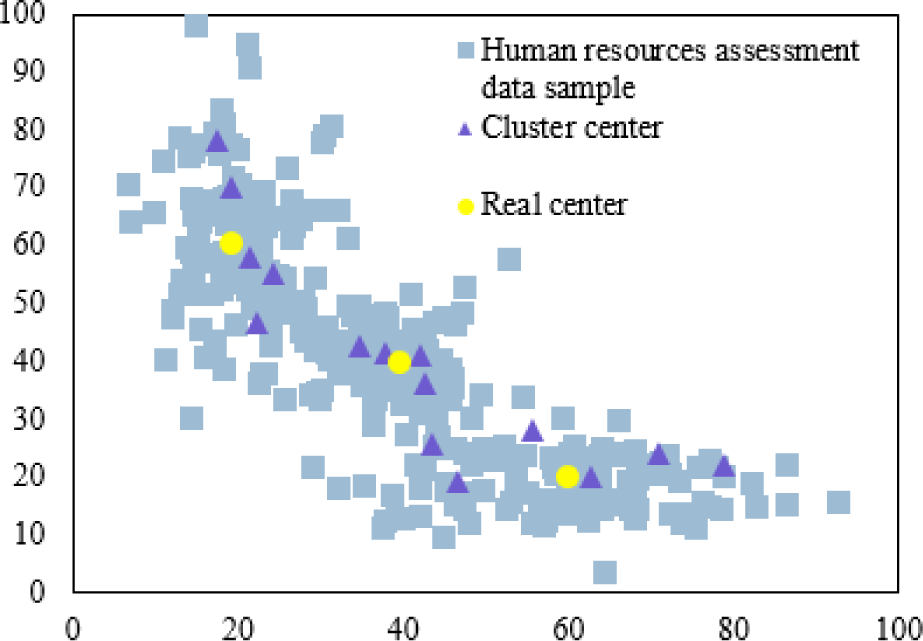Fig. 5. Data points and distribution of clustering center.

After several iterations, the clustering results are shown in Fig. 6. Among them, Fig. 6(a) shows that after two iterations, 10 of the 15 initial clustering centers remain; Fig. 6(b) shows that after five iterations, there are 6 cluster centers left; Fig. 6(c) shows that after nine iterations, there are 5 cluster centers left; Fig. 6(d) shows that after 12 iterations, there are 3 cluster centers left, and the number of cluster centers does not change in subsequent iterations. Therefore, a good clustering effect can distinguish dense and sparse areas in the object space, which is helpful for human resource assessment.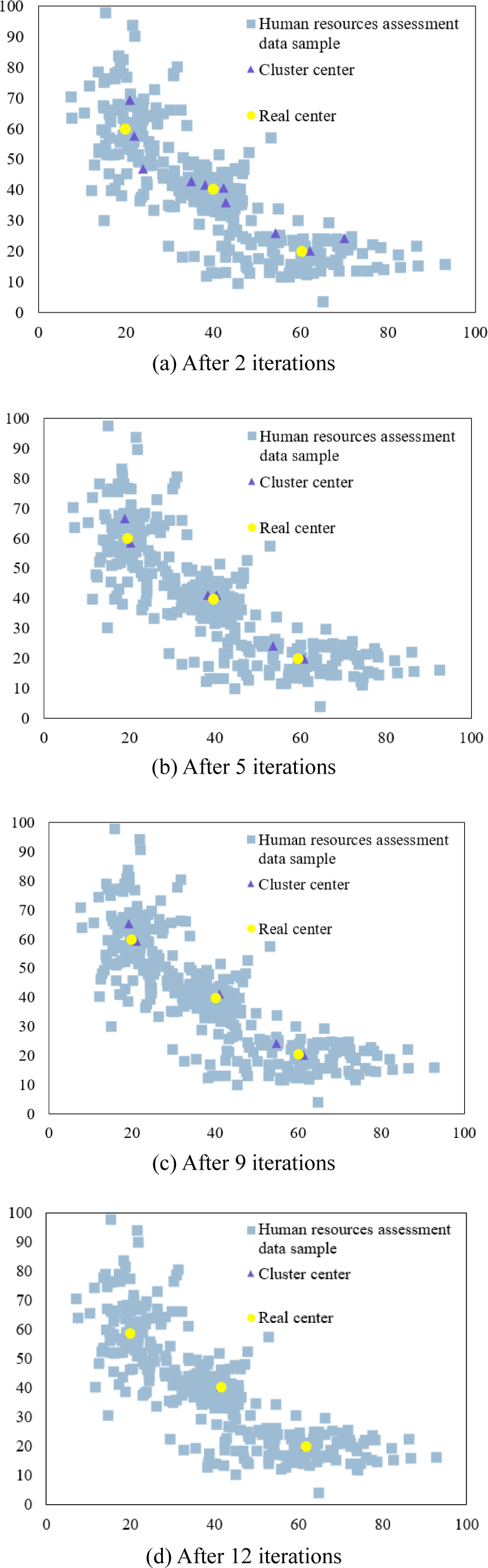Fig. 6. Clustering results.

Five different groups of real cluster centers were set for the experiment. Each data group is generated the same way as in the previous test, by specifying the corresponding cluster centers and generating random data samples based on different covariance matrices. The mutual information index is used to evaluate the accuracy of clustering. The mutual information index marks the accuracy of correct classification by calculating the average mutual information size after matching the clustering results with the actual classification, defined as follows.

$\text{NMI}=\frac{{\sum }_{p=1}^{c}{\sum }_{q=1}^{c}{n}_{pq}\text{log}\left(\frac{N{n}_{pq}}{{n}_{p}{n}_{q}}\right)}{\sqrt{{\sum }_{p=1}^{c}{n}_{p}\text{log}\left(\frac{{n}_{p}}{N}\right){\sum }_{q=1}^{c}{n}_{q}\text{log}\left(\frac{{n}_{q}}{N}\right)}},$
(26)

where npq is the number of data whose real class is p and is divided into clustering result q, np is the number of data whose clustering result is p, nq is the number of data whose true class is q, N is the total number of data points. The NMI of different cluster centers is shown in Table 2.

Table 2. Clustering mutual information index data.
No. Mean Variance
1 0.9221 0.0001
2 0.9042 0.0006
3 0.9323 0.0001
4 0.9246 0.0012
5 0.9121 0.0002

Table 2 shows that the clustering algorithm has high accuracy for data clustering. The real clustering center does not affect the clustering results, so the algorithm can automatically cluster data samples in various situations.

The different initial numbers of cluster centers are selected for operation, and the change in the number of cluster centers in the iteration is shown in Fig. 7. Fig. 7 shows that although the initial number of cluster centers selected is different, the number of cluster centers is decreasing in the iteration process. After several iterations, the number of cluster centers is stable at three. Therefore, the clustering algorithm is not sensitive to the initial number of cluster centers change and can reliably converge to a reasonable number of cluster centers.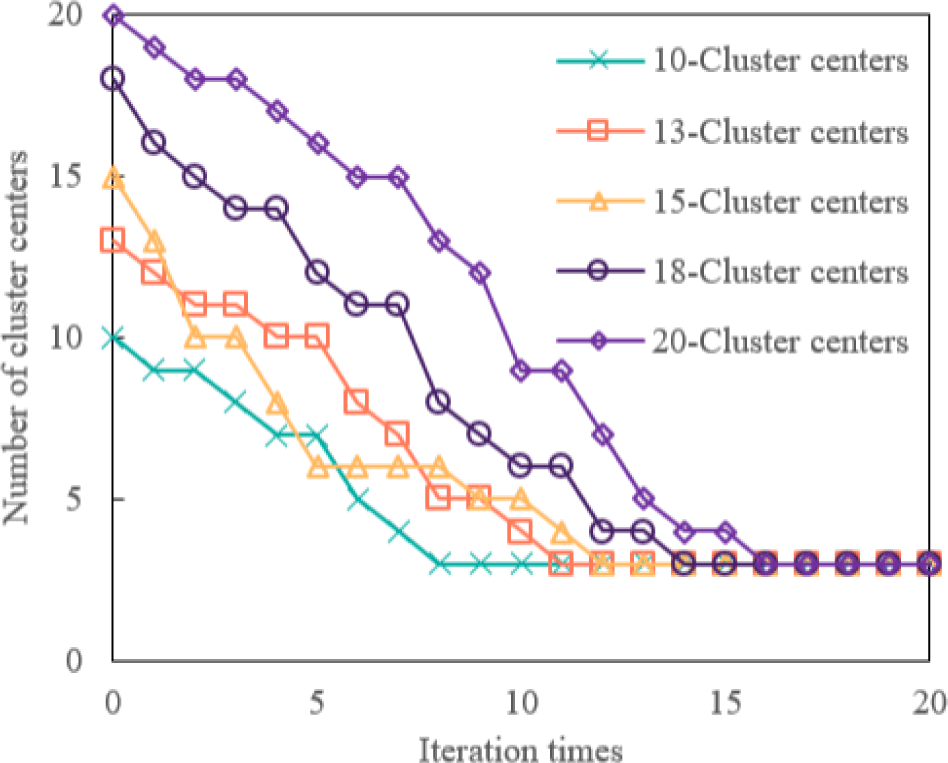Fig. 7. Number of clustering center in operation process.

## VI. CONCLUSION

This paper studies the IoT-driven human resources assessment optimization mechanism. We propose using DRQN to memorize previous environmental state information to solve the problem of partially observable multi-IoT node task offloading. The simulation results demonstrate that DRQN has excellent energy consumption, cost, and latency performance, showing that after real-time processing, the data gathered by IoT nodes can meet the requirements of human resource assessment. Moreover, this study combines the soft subspace and fuzzy clustering algorithms based on the competitive merging mechanism. It proposes a fuzzy weighting clustering algorithm based on the soft subspace combined with the competitive merging mechanism. The simulation results show that the algorithm can quickly realize the clustering analysis of data samples. The calculation results are independent of the number of initial data clusters and the location of the initial cluster center.

Based on this study, the role of bidirectional DQN, prioritized experience replay, and generative adversarial network in the practical application of edge computing can be further studied. With new AI technologies, decision-making algorithms have transformed from rules to shallow supervised learning, deep learning, and deep reinforcement learning, from single-scenario optimization to joint optimization of offloading decision-making and resource allocation. However, many things could still be improved in the existing methods. In the future, cooperation and collaboration between multiple IoT nodes will become the mainstream development trend, mutual competition, internal friction will be continuously reduced, and hybrid optimization will create higher value.

## REFERENCES

.

O. Yabanci, "From human resource management to intelligent human resource management: A conceptual perspective," Human-Intelligent Systems Integration, vol. 1, pp. 101-109, Dec. 2019..

S. Zheng, G. Zhu, J. Zhang, and W. Feng, "Towards an adaptive human-centric computing resource management framework based on resource prediction and multi-objective genetic algorithm," Multimedia Tools and Applications, vol. 76, pp. 17821-17838, Sep. 2017..

R. Das, "Paradigm development and diffusion in human resource management over 39 years of scholarship," Employee Responsibilities and Rights Journal, vol. 35, pp. 3-28, Mar. 2023..

F. Amjad, W. Abbas, M. Zia-UR-Rehman, S. A. Baig, M. Hashim, and A. Khan, et al., "Effect of green human resource management practices on organizational sustainability: The mediating role of environmental and employee performance," Environmental Science and Pollution Research, vol. 28, pp. 28191-28206, Jun. 2021.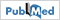.

B. Ferreira, C. Curado, and M. Oliveira, "The contribution of knowledge management to human resource development: A systematic and integrative literature review," Journal of the Knowledge Economy, vol. 13, pp. 2319-2347, Sep. 2022..

Y. Liu, W. Zhang, Q. Zhang, and M. Norouzi, "An optimized human resource management model for cloud-edge computing in the internet of things," Cluster Computing, vol. 25, pp. 2527-2539, Aug. 2022..

X. Chu, H. Jiang, B. Li, D. Wang, and W. Wang, "Editorial: Advances in mobile, edge and cloud computing," Mobile Networks and Applications, vol. 27, pp. 219-221, Feb. 2022..

C. Fan, "Research on the structural optimization of the data mining-based enterprise human resource management," Journal of The Institution of Engineers (India): Series C, vol. 103, pp. 931-938, Aug. 2022..

Y. Zhong, L. Chen, C. Dan, and A. Rezaeipanah, "A systematic survey of data mining and big data analysis in internet of things," The Journal of Supercomputing, vol. 78, pp. 18405-18453, Nov. 2022..

R. Dijkman, J. Gao, A. Syamsiyah, B. van Dongen, P. Grefen, and A. T. Hofstede, "Enabling efficient process mining on large data sets: Realizing an in-database process mining operator," Distributed and Parallel Databases, vol. 38, pp. 227-253, Mar. 2020..

L. Jian, "Design of enterprise human resources decision support system based on data mining," Soft Computing, vol. 26, pp. 10571-10580, Oct. 2020..

X. Ran, Y. Xi, Y. Lu, X. Wang, and Z. Lu, "Comprehensive survey on hierarchical clustering algorithms and the recent developments," Artificial Intelligence Review, vol. 56, pp. 8219-8264,, Dec. 2022..

C. Sharma, S. Sakhuja, and S. Nijjer, "Recent trends of green human resource management: Text mining and network analysis," Environmental Science and Pollution Research, vol. 29, pp. 84916-84935, Dec. 2022..

J. Zhang and Z. Chen, "Exploring human resource management digital transformation in the digital age," Journal of the Knowledge Economy, DOI:, Mar. 2023..

C. Zhang, "Evaluation and analysis of human resource management mode and its talent screening factors based on decision tree algorithm," The Journal of Supercomputing, vol. 78, pp. 15681-15713, Sep. 2022..

S. Bankins, P. Formosa, Y. Griep, and D. Richards, "AI decision making with dignity? contrasting workers’ justice perceptions of human and AI decision making in a human resource management context," Information Systems Frontiers, vol. 24, pp. 857-875, Jun. 2022..

S. Ren, G. Tang, and S. Jackson, "Green human resource management research in emergence: A review and future directions," Asia Pacific Journal of Management, vol. 35, pp. 769-803, Sep. 2018..

A. Naghib, N. Jafari Navimipour, M. Hosseinzadeh, and A. Sharifi, "A comprehensive and systematic literature review on the big data management techniques in the internet of things," Wireless Networks, vol. 29, pp. 1085-1144, Apr. 2023..

E. Adi, A. Anwar, Z. Baig, and S. Zeadally, "Machine learning and data analytics for the IoT," Neural Computing and Applications, vol. 32, pp. 16205-16233, Feb. 2020..

D. S. Park, "Future computing with IoT and cloud computing," The Journal of Supercomputing, vol. 74, pp. 6401-6407, Dec. 2018..

P. Singh and R. Singh, "Energy-efficient delay-aware task offloading in fog-cloud computing system for IoT sensor applications," Journal of Network and Systems Management, vol. 30, p. 14, Oct. 2021..

X. Ma, A. Zhou, S. Zhang, Q. Li, A. Liu, and S. Wang, "Dynamic task scheduling in cloud-assisted mobile edge computing," IEEE Transactions on Mobile Computing, vol. 22, pp. 2116-2130, Apr. 2023..

C. Zhang and H. Du, "DMORA: Decentralized multi-SP online resource allocation scheme for mobile edge computing," IEEE Transactions on Cloud Computing, vol. 10, pp. 2497-2507, Jan. 2023..

Y. Cui, Y. Liang, and R. Wang, "Resource allocation algorithm with multi-platform intelligent offloading in D2D-enabled vehicular networks," IEEE Access, vol. 7, pp. 21246-21253, Mar. 2019..

L. Dong, W. Wu, Q. Guo, M. N. Satpute, T. Znati, and D. Du, et al., "Reliability-aware offloading and allocation in multilevel edge computing system," IEEE Transactions on Reliability, vol. 70, pp. 200-211, Apr. 2021..

W. Chen, D. Wang, and K. Li, "Multi-user multi-task computation offloading in green mobile edge cloud computing," IEEE Transactions on Services Computing, vol. 12, pp. 726-738, Sep. 2020..

H. Zhao, J. Geng, and S. Jin, "Performance research on a task offloading strategy in a two-tier edge structure-based MEC system," The Journal of Supercomputing, vol. 79, pp. 10139-10177, Sep. 2023..

I. A. Elgendy, W. Z. Zhang, H. He, B. B. Gupta, and A. Abd El-Latif, "Joint computation offloading and task caching for multi-user and multi-task MEC systems: Reinforcement learning-based algorithms," Wireless Networks, vol. 27, pp. 2023-2038, Apr. 2021..

A. A. Ateya, A. Muthanna, A. Koucheryavy, Y. Maleh, and A. Abd El-Latif, "Energy efficient offloading scheme for MEC-based augmented reality system," Cluster Computing, vol. 26, pp. 789-806, Feb. 2023..

X. Li, "A computing offloading resource allocation scheme using deep reinforcement learning in mobile edge computing systems," Journal of Grid Computing, vol. 19, p. 35, Jul. 2021..

P. Agarwal, S. Mehta, and A. Abraham, "A meta-heuristic density-based subspace clustering algorithm for high-dimensional data," Soft Computing, vol. 25, pp. 10237-10256, Feb. 2021..

Y. Guo, R. Wang, J. Zhou, Y. Chen, H. Jiang, and S. Han, et al., "Soft subspace fuzzy clustering with dimension affinity constraint," International Journal of Fuzzy Systems, vol. 24, pp. 2283-2301, Jul. 2022..

M. S. Kumar, I. Gupta, S. K. Panda, and P. K. Jana, "Granularity-based workflow scheduling algorithm for cloud computing," The Journal of Supercomputing, vol. 73, pp. 5440-5464, Dec. 2017..

Z. Zhou, L. Lai, and Y. Dong, "Quantification of value of information associated with optimal observation actions within partially observable markov decision processes," KSCE Journal of Civil Engineering, vol. 26, pp. 5173-5186, Dec. 2022..

T. Mumtaz, S. Muhammad, M. I. Aslam, and I. Ahmed, "Inter-slice resource management for 5G radio access network using markov decision process," Telecommunication Systems, vol. 79, pp. 541-557, Apr. 2022..

S. A. Serrano, E. Santiago, J. Martinez-Carranza, E. F. Morales, and L. E. Sucar, "Knowledge-based hierarchical POMDPs for task planning," Journal of Intelligent & Robotic Systems, vol. 19, p. 82, Apr. 2021..

N. Le, V. S. Rathour, K. Yamazaki, K. Luu, and M. Savvides, "Deep reinforcement learning in computer vision: A comprehensive survey," Artificial Intelligence Review, vol. 55, pp. 2733-2819, Apr. 2022.## AUTHORSQiong Wu received her Bachelor Degree at Changchun University of Technology in 2005 and received the Master’s degree at Changchun University of Technology in 2009. She is currently an assistant research fellow at Changchun University of Technology. Her research interests include human resource management, strategic management and management of technology. E-mail: wuqiong@ccut.edu.cn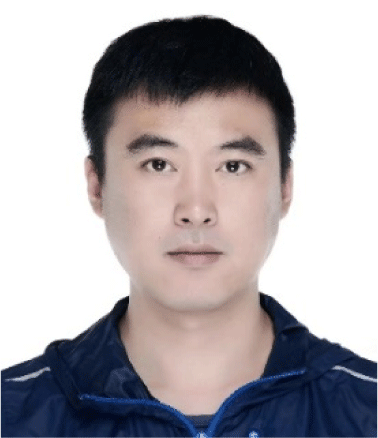Lijun Chen received his Bachelor Degree at Changchun University of Technology in 2006 and received the Master’s degree at Changchun University of Technology in 2019. He is currently an assistant research fellow at Changchun University of Technology. His research interests include human resource management, design and development of information system and data analysis. E-mail: chenlijun@ccut.edu.cn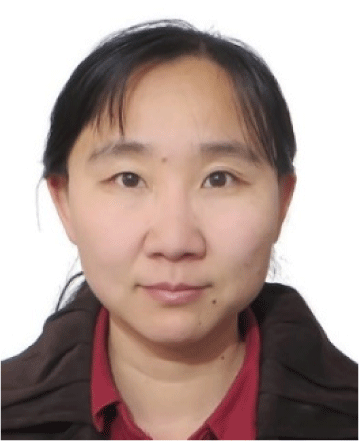Ying Shen received her Bachelor Degree at Tianjin University in 1999 and received her Master Degree at Changchun University of Technology in 2004 and received her Doctor Degree at Jilin University in 2007. She is currently a professor at Changchun University of Technology . Her research interests including technological innovation management and human resource management.